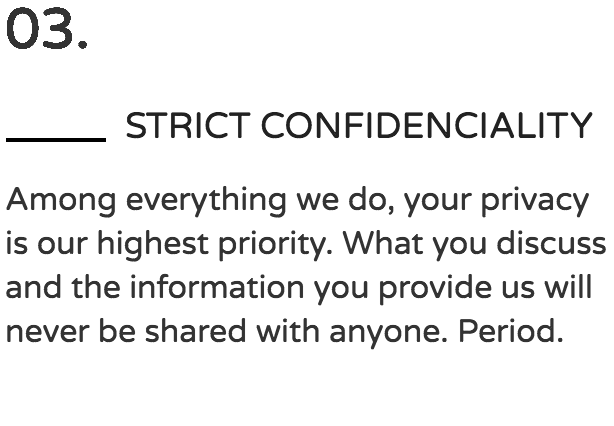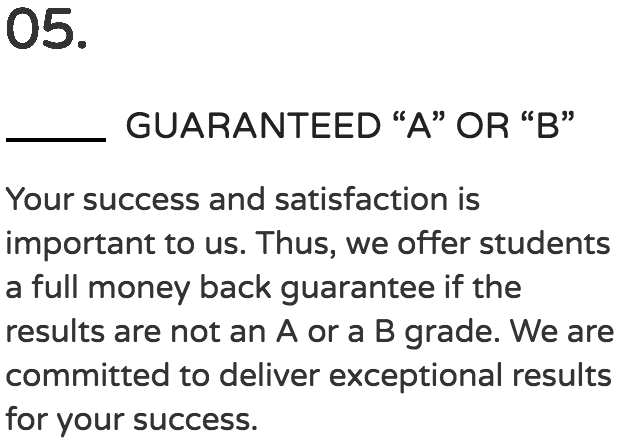Your

# Quiz, Test, Homework, Class

## DONE.

#### Online class help, right now.

100% Confidential
No Spam
We will never spam you. We need your phone number to provide our services. By clicking on the Request Callback button, you agree to our Terms of Use, Privacy and Cookie Policies.

What Are The Benefits Of Using Our Services?# Revolutionize How You Study College Algebra with These 10 Easy Tips

Most students struggle with algebra in colleges because they are trying to memorize. But when an algebra problem changes so do the steps; as such, leaving students confused. You need to worry no more because the key to understanding algebra is to comprehend each step. We understand algebra is not a walk in the park. However, with the right explanation and demonstration, you can manage to understand and pass algebra with ease. Below we have formulated 10 studying tips that quickly help in understanding college algebra.

## 1. Understand Basic Arithmetic

statistic assignment help

First, it is important to have an understanding of basic arithmetic of addition, subtraction, multiplication, and division. We know this is elementary school mathematics but algebra relies on these concepts. Knowing simple arithmetic helps one to understand the concept behind an operation.

For example, 3* 4 = 12, this is also equal to 3+3+3+3 = 12. As such, it is essential to understand a concept that multiplying 3 and 4 is actually adding 3 four times.

## 2. Put the focus on PEMDAS

pay someone to do my coursework

PEMDAS is the abbreviation for Parentheses, Exponents, Multiplication, Division, Addition, and Subtraction. It is important to remember PEMDAS while studying algebra. Some students use this mnemonic “Please Excuse My Dear Aunt Sally.” It demonstrates how one should deal with the problem; as a start to deal with parentheses, then exponents followed by multiplication, addition, and subtraction from left to right.

For Example, 10+ (4 ÷ 2 x 2)2 – 12 + 14

Utilizing PEMBDAS will begin with expression in the parentheses. We will calculate first by dividing 4/2 then multiplying by 2

10+ (2 x 2)2 – 12 + 14

10+ (4)2 – 12 + 14

Now we are done with parentheses and then we work on exponent, i.e., (4)2:

10 + 16 – 12 +14

Now we do addition and finalize by subtracting,

In terms of Algebra here is an example,

## 3. Be Cautious about Negative and Positive Numbers

Often students don’t have a problem with positive integers; as such negative numbers causes them a problem. Therefore, a negative number is a number with a negative sign in front of it.

It is essential in algebra to be mindful of the sign, as a negative sign has an impact when adding, subtracting, dividing or multiplying. The below are examples demonstrating an outcome with a negative when divided, multiplied and added by a negative or positive number:

-3 – 5 = -3 + (-5) = -8

Negative minus negative = negative

-10 + 7 = -3

-10+13=+3

Negative plus positive = either positive or negative

When we multiply and divide two negative numbers we get a positive number. However, when we divide or multiply positive with negative we get a negative number.

## 4. Follow Each Step meticulously

Paying attention to signs and every step will help ensure that you arrive at your answers. Following each step will allow you to keep your work organized and free from small errors. As well, it allows getting credit even if your final answer is wrong.

For example,

## 5. Don’t Be Afraid of Letters

We know that you have learned that math is about numbers but in algebra you starting to wonder about x’s and y’s. These letters are known as variables and they are numbers too (because they represent a number in a mathematical function).

For example, x= 3+2

Therefore, we need to solve for x =6.

As such, having more variables implies that there are more things to find and solve. But it is important to find one variable at a time.

## 6. Understand Formulas

Formulas are the most helpful in tackling algebra. Based on the class you are in you must understand how concepts are applied. Memorizing can help if you understand what each variable in a formula stands for.

The examples of the most common formulas in algebra are:

## 7. Practice! Practice Makes Perfect

To ensure that you understand algebra there is a need to practice with as many problems as possible. Practice with classmates and on your own. This gives you a chance to learn from the mistakes. As well, it helps you familiarize yourself with formulas.

## 8. Never Divide By Zero

In Mathematical Bible, there is a rule that states that “Thou shall not divide thee by zero” because you will never be able to do it and no one can. Equation and functions are equated to zero in algebra, never think for a moment you can divide by zero.

## 9. Be Careful of the Parentheses & Exponent

Parentheses are a very important component in algebra; as such they should be treated with a lot of mindfulness.

Below is an example of a problem that helps to demonstrate the significance of the parentheses:

Consider (-2)2 and -22

The number in parentheses implies we are going to multiply -2*-2 = 9; whereas, -22 implies that we going to multiply -2*2 = -9.

## 10. Be Mindful of Distributions & Only Cancel Factors

This is our last tip but it does not mean it the least important. In fact, it is essential in algebra to be mindful of your distribution to avoid common mistakes. Distributions and factoring are present in almost every equation that you will ever solve. Without knowing the bases of distributions and factoring there is no way you can possibly be successful or even pass algebra for that matter.

We Accept:Still Got Questions?

Real Customer Reviews

View All

Latest Articles### College Blog Posts You Should Read This Pandemic### Best Vitamins and Supplements for College Students### DONE! Pay someone to do math homework for you – Get Help

Get in Touch

You will find yourself working with a team who will stop at nothing to help you succeed.

Text Us

+1 (248) 220-7202

Live Chat

GET STARTED